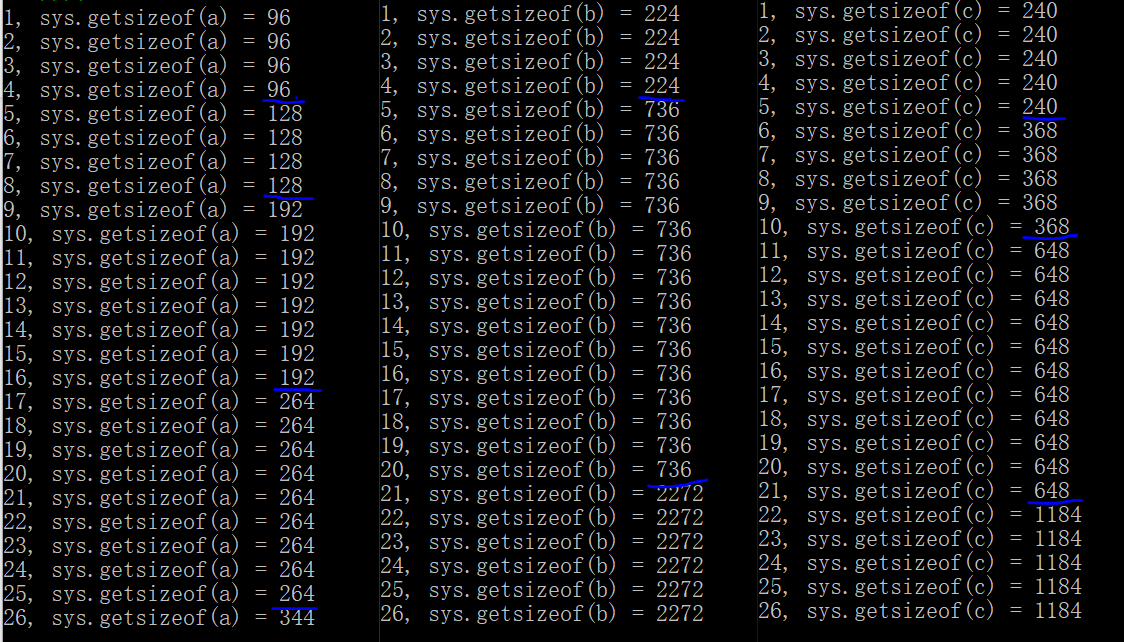# Python 的内置对象都藏了哪些小秘密？

Python 中的`sys` 模块极为基础而重要，它主要提供了一些给解释器使用（或由它维护）的变量，以及一些与解释器强交互的函数。

• 该方法用于获取一个对象的字节大小（bytes）
• 它只计算直接占用的内存，而不计算对象内所引用对象的内存

``````import sys

a = [1, 2]
b = [a, a]  # 即 [[1, 2], [1, 2]]

# a、b 都只有两个元素，所以直接占用的大小相等
sys.getsizeof(a) # 结果：80
sys.getsizeof(b) # 结果：80
``````## 1、空对象不是“空”的！

``````import sys
sys.getsizeof("")      # 49
sys.getsizeof([])      # 64
sys.getsizeof(())      # 48
sys.getsizeof(set())   # 224
sys.getsizeof(dict())  # 240

# 作为参照：
sys.getsizeof(1)       # 28
sys.getsizeof(True)    # 28
``````

## 2、内存扩充不是均匀的！

``````import sys
letters = "abcdefghijklmnopqrstuvwxyz"

a = []
for i in letters:
a.append(i)
print(f'{len(a)}, sys.getsizeof(a) = {sys.getsizeof(a)}')

b = set()
for j in letters:
print(f'{len(b)}, sys.getsizeof(b) = {sys.getsizeof(b)}')

c = dict()
for k in letters:
c[k] = k
print(f'{len(c)}, sys.getsizeof(c) = {sys.getsizeof(c)}')
``````• 超额分配机制： 申请新内存时并不是按需分配的，而是多分配一些，因此当再添加少量元素时，不需要马上去申请新内存
• 非均匀分配机制： 三类对象申请新内存的频率是不同的，而同一类对象每次超额分配的内存并不是均匀的，而是逐渐扩大的

## 3、列表不等于列表！

``````# 静态创建对象
set_1 = {1, 2, 3, 4}
set_2 = {1, 2, 3, 4, 5}
dict_1 = {'a':1, 'b':2, 'c':3, 'd':4, 'e':5}
dict_2 = {'a':1, 'b':2, 'c':3, 'd':4, 'e':5, 'f':6}

sys.getsizeof(set_1)  # 224
sys.getsizeof(set_2)  # 736
sys.getsizeof(dict_1) # 240
sys.getsizeof(dict_2) # 368
``````

``````list_1 = ['a', 'b']
list_2 = ['a', 'b', 'c']
list_3 = ['a', 'b', 'c', 'd']
list_4 = ['a', 'b', 'c', 'd', 'e']

sys.getsizeof(list_1)  # 80
sys.getsizeof(list_2)  # 88
sys.getsizeof(list_3)  # 96
sys.getsizeof(list_4)  # 104
``````## 4、消减元素并不会释放内存！

``````import sys
a = [1, 2, 3, 4]
sys.getsizeof(a) # 初始值：96
a.append(5)      # 扩充后：[1, 2, 3, 4, 5]
sys.getsizeof(a) # 扩充后：128
a.pop()          # 缩减后：[1, 2, 3, 4]
sys.getsizeof(a) # 缩减后：128
``````## 5、空字典不等于空字典！

``````import sys
a = [1, 2, 3]
b = {1, 2, 3}
c = {'a':1, 'b':2, 'c':3}

sys.getsizeof(a) # 88
sys.getsizeof(b) # 224
sys.getsizeof(c) # 240

a.clear()        # 清空后：[]
b.clear()        # 清空后：set()
c.clear()        # 清空后：{}，也即 dict()
``````

``````# 承接前面的清空操作：
sys.getsizeof(a) # 64
sys.getsizeof(b) # 224
sys.getsizeof(c) # 72
``````Python教程：如何用xlrd和xlwt库读和写Excel表格？
Python安装MySQL-python：EnvironmentError的解决办法